# Bunching estimation with bunchr

#### 2016-12-02

A growing literature in empirical economics is focused on bunching. Loosely speaking, bunching occurs when many people self select to a specific location in the range of some variable. When this happens, a histogram of this variable might show a visible “bunch” - a large mass that would not be otherwise predicted by the surrounding bins. This bunching behavior is then interpreted as the response to some economic reality. In words of someone smarter than me:

“This approach uses bunching around points that feature discontinuities in incentives to elicit behavioral responses and estimate structural parameters.” 1

With the increasing use of administrative data, bunching has been used mostly by public and labor economists. Two main types of bunch-inducing setting are explored. First, where budget sets suddenly change slopes, creating a point where budget sets are continuous but non-differentiable. These “kinks” in budget sets have been explored in the case of Earned Income Tax Credits in the US2 and income tax rates in Denmark3. The second type are notches, where a policy sets a discontinuity in the budget set. For example, bunching in earnings of individuals is observed in Pakistan, where the average tax rates, rather than the marginal ones, change when earnings cross thresholds4. This creates a drop in the budget line of individuals, as all earnings are subject to a higher tax rate once earnings exceed the threshold.

When a policy introduces a kink or a notch, a structural analysis can be used to estimate a parameter of interest, such as the elasticity of earnings with respect to marginal tax rates. To do this, a specific function must be assumed for the agents. In many cases, a convenient function for analysis is quasi-linear and iso-elastic.

## What can you do with bunchr?

NEW (Dec. 2016)! Explore bunching simulations in an interactive interface, using the bunchApp. After loading bunchr, run bunchApp() and start playing.

The bunchr package can be used to visualize bunching, calculate counter-factual distributions, and estimate the compensated elasticity of earnings w.r.t. marginal tax rates, as usually done in the literature cited above. There is also a function to simulate earnings given certain parameters. bunchr’s language is of earnings and elasticities of earnings, but can be used for other suitable bunching analysis. Indeed, you can provide a title and x-axis label for the output plots.

Remember that, as usual, more observations are better. Bunching analysis involves the creation of a histogram, similar to a density curve. The narrower the bins of this histogram, the better. Wider bins could bias elasticity estimates. For a kink analysis, the elasticity formula takes into account the extra bunching over the width of the excluded area. Setting bins too wide could attenuate the estimates. For notches, the procedure involves finding the “end” of the notch, where the discontinuity in incentives is no longer relevant. This limit, referred to as delta_zed in the code, is determined in number of bins after the bunching bin. Setting bins too wide limits the set of potential delta_zed, which might bias the estimates.

Having more observations, one can use narrower bins and still avoid a “jumpy” looking histogram. It is better to have a smoother looking histogram, as the counter-factual histogram for the area of kink or notch is made by interpolation from the area outside of the notch. Smoother histograms make more reliable counter-factuals. As a robustness check for any analysis, I recommend comparing the results of analysis with varying bin widths.

## Pre-analysis: Viewing a distribution

All you need is a vector of earnings. Using bunch_viewer, you can see how a histogram of your data looks like. Additionally, you can see where you could potentially set some of the parameters for analysis. And, you can save the histogram as an R histogram object.

Let’s create an earning distribution for a kinked budget set. First, I create a vector of latent abilities, with 100,000 values. Then, I simulate the earnings for a situation where everyone’s elasticity of earnings w.r.t. marginal tax rate is 0.2 (elas = 0.2). The simulation includes a kink at the earning point of 1000 (zstar = 1000), where the marginal tax rate increases from 0% to 10% (t1 = 0, t2 = 0.1), the notch height is zero (Tax = 0). After creating the earning vector, we can view it with bunch_viewer.

As a preparation for further analysis, I can specify where the counter-factual distribution will be calculated (setting cf_start and cf_end), and where the bunching seems to be (exclude_before and exclude_after) relative to the place of the notch. Note that these are stated in number of bins. The wider the bins, the greater the distances from the kink! That’s where bunch_viewer comes handy.

In this case, one can observe the whole bunching bin and the rest of the distribution quite well. In other cases, specially notches (where bunching is more substantial), the height of the bunching bin can be so high that, in order to include it in the graph, the rest of the distribution can hardly be seen. In these cases, I would keep the default option of “trimming” the vertical axis by keeping the default options and not specifying trimy = F. Also note that you can personalize the plot’s title and x-axis label.

set.seed(42)
ability_vec <- 4000 * rbeta(100000, 2, 5)
earning_vec <- sapply(ability_vec, earning_fun, 0.2, 0, 0.1, 0, 1000)
bunch_viewer(earning_vec, 1000, cf_start = 10, cf_end = 10, exclude_before = 2,
exclude_after = 2, binw = 50, trimy=F)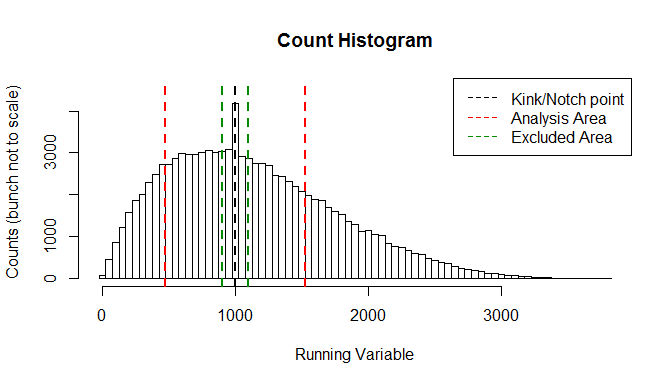## Analyzing a kink

We viewed the distribution. Now we want to get an estimate for the elasticity of earnings. We can use the same specification as we did while viewing. Note that, in order to calculate the elasticity, we must provide the marginal tax rates!

kink_est <- bunch(earning_vec, zstar = 1000, t1 = 0, t2 = 0.1, Tax = 0,
cf_start = 10, cf_end = 10,
exclude_before = 2, exclude_after = 2, binw = 50, nboots = 100)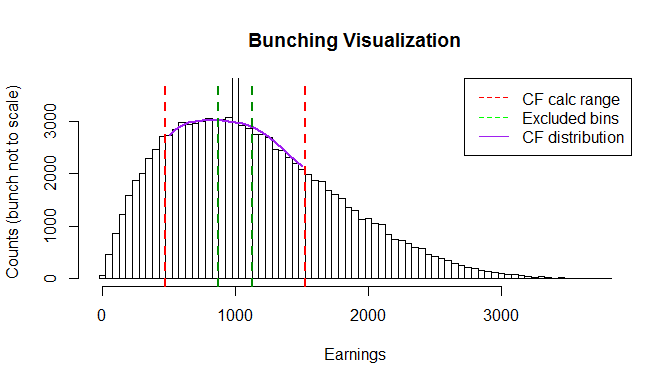kink_est$e ##  0.186233 quantile(kink_est$booted_e, probs=c(0, 0.05, 0.1, 0.5, 0.9, 0.95, 1))
##         0%         5%        10%        50%        90%        95%
## 0.09330628 0.11666582 0.13313885 0.18567137 0.23956820 0.25130516
##       100%
## 0.32462423
kink_est$Bn ##  1170.216 quantile(kink_est$booted_Bn, probs=c(0, 0.05, 0.1, 0.5, 0.9, 0.95, 1))
##        0%        5%       10%       50%       90%       95%      100%
##  599.0476  744.2115  851.2940 1166.8193 1481.8489 1541.6775 1939.3720

Note: we modeled the earnings with elasticity of 0.2, but got an estimate of 0.186. That’s no so bad, considering that the width of the bins and the excluded area have an attenuating effect. We might not be very happy with the bootstrapped confidence interval we got. It’s pretty wide, both for the elasticity estimate and the amount of bunching. Since the number of observations allows it, and the simulation is pretty “clean”, we can try this with narrower bins (width 1 instead of 50), and excluded area (same number of bins, but bins are narrower!). This does shrink the confidence intervals, and gives a point estimate closer to the value we used to generate the data. Also, the plot title and x-axis label can be modified by the user. In this case, however, plotting is suppressed.

kink_est <- bunch(earning_vec, zstar = 1000, t1 = 0, t2 = 0.1, Tax = 0,
cf_start = 500, cf_end = 500,
exclude_before = 10, exclude_after = 10, binw = 1,
nboots = 100, seed = 123, draw = F)
kink_est$e ##  0.1932915 quantile(kink_est$booted_e, probs=c(0, 0.05, 0.1, 0.5, 0.9, 0.95, 1))
##        0%        5%       10%       50%       90%       95%      100%
## 0.1770582 0.1802953 0.1834727 0.1915006 0.2035105 0.2061229 0.2116499
kink_est$Bn ##  1223.928 quantile(kink_est$booted_Bn, probs=c(0, 0.05, 0.1, 0.5, 0.9, 0.95, 1))
##       0%       5%      10%      50%      90%      95%     100%
## 1127.625 1143.042 1164.198 1219.175 1284.605 1304.872 1332.322

## Analyzing a notch

First, let’s create an earning distribution with a notch. This time, I will use bunch_viewer without trimming the vertical axis. Now, the excluded area is where the notch seems to be taking place. I want to make sure I have enough area inside the red lines (where counter-factual is calculated) and outside the green lines (the excluded area). Of course, in this particular case we could just set the red lines at both ends of the histogram. In other cases, however, we might want to limit the analysis area (e.g. there are other notches or kinks).

It’s best to set the cf_end variable so it lays to the right of what seems to be the end of the effect of the notch, but not too much to the right. The function uses the area between the red and green lines to calculate an initial counter-factual distribution, so you want to make it easy by having quite a few bins there. I would refrain from including areas with idiosyncratic density patterns in the counter-factual area, as it might make the interpolation harder and less accurate.

Basically, what we need to calculate the elasticity is the size of the notch, or $$\Delta z^*$$. While this is clear in this case, where elasticity is heterogeneous it might not be so clear cut. The process goes like this: a counter-factual histogram is created. Then, the extra bunching (sum differences between the actual and counter- factual histograms from the beginning of the excluded area to the notch point) is calculated. That bunching comes from the right side of the histogram. Running on the bins to the right of the notch bin, the differences between the counter-factual and real histogram are added to the initial bunching sum (they should be negative). When the sum hits zero, the process stops and the current bin is declared the end of the notch. $$\Delta z^*$$ is the distance from the center of that bin to the notch point.

Notch analysis takes a little longer, mainly because of an iterative process in searching for the end of the notch.

earning_vec <- sapply(ability_vec, earning_fun, 0.2, 0.1, 0.1, 200, 1000)
bunch_viewer(earning_vec, 1000, 20, 50, 2, 25, binw = 20)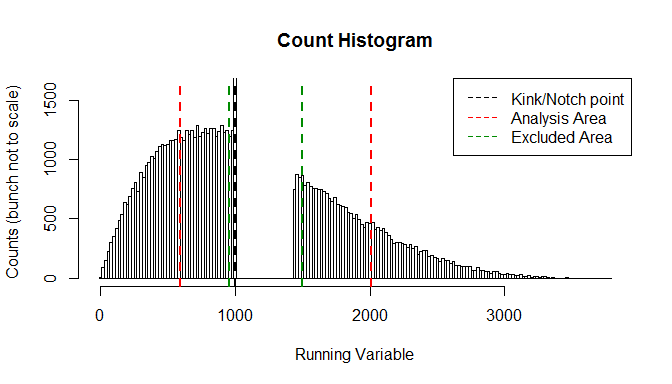notch_est <- bunch(earning_vec, zstar = 1000, t1 = 0.1, t2 = 0.1, Tax = 200,
cf_start = 20, cf_end = 50, force_after = FALSE,
exclude_before = 2, exclude_after = 25, binw = 20,
nboots = 100, seed = 123)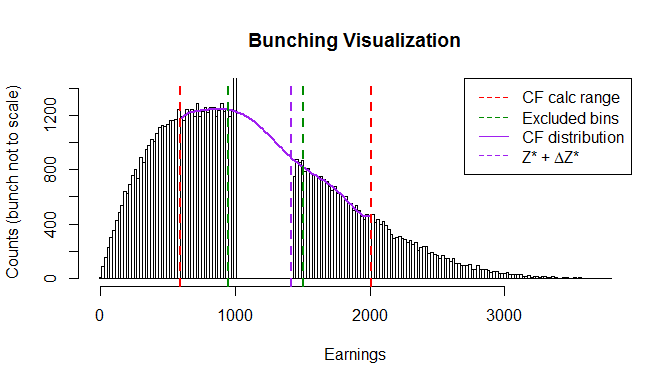notch_est$e ##  0.1843316 quantile(notch_est$booted_e, probs=c(0, 0.05, 0.1, 0.5, 0.9, 0.95, 1))
##        0%        5%       10%       50%       90%       95%      100%
## 0.1203677 0.1407937 0.1621033 0.2074509 0.2074509 0.2074509 0.2074509

The example above is a good one: _delta_zed seems to be the actual end of the notch. Sometimes, the output plot will show $$\Delta z^*$$ not concurring with what visibly seems like the end of the notch. This usually means that the elasticity estimates will be wrong. In that case, it might be useful to change the exclude_after parameter and see if the plot come out better.

Another option is using the force_after option to set the end of the notch manually. However, this means that not all bunching comes from inside the notch. When using this option, make sure to understand exactly what is the parameter you are estimating.

Another issue is the counter-factual distribution. For larger notches, the interpolation is harder. Remember the interpolation is based on the histogram bins outside the excluded area (green lines) but inside the analysis area (red). If the counter-factual distribution in the output plot does not seem convincing, a few measures can be taken:

• Widen the counter-factual analysis area (red lines).
• Change the order of polynomial used to construct the counter-factual.
• Change the option of model selection for counter-factual.

As for the distribution of bootstrapped estimates, note that it might be less “smooth” than in the kink analysis. Recall that the major change in the different runs is the estimated number of bins forming delta_zed. As these are integers, the distribution of estimates will not be very smooth. With narrower bins, there should be more potential values for the elasticity estimates.

## The Importance of Sample Size

How meaningful is a having a large sample size? This demonstration shows some of the effects of sample size on kink estimations. First, I’ll use a small earning vector: 10,000 observations in total. The confidence interval is quite large.

ability_vec_small <- 4000 * rbeta(10000, 2, 5)
earnings_small <- sapply(ability_vec_small, earning_fun, 0.2, 0, 0.1, 0, 1000)
kink_est <- bunch(earnings_small, zstar = 1000, t1 = 0, t2 = 0.1, Tax = 0,
cf_start = 10, cf_end = 10,
exclude_before = 1, exclude_after = 1, binw = 50,
nboots = 100, seed = 123)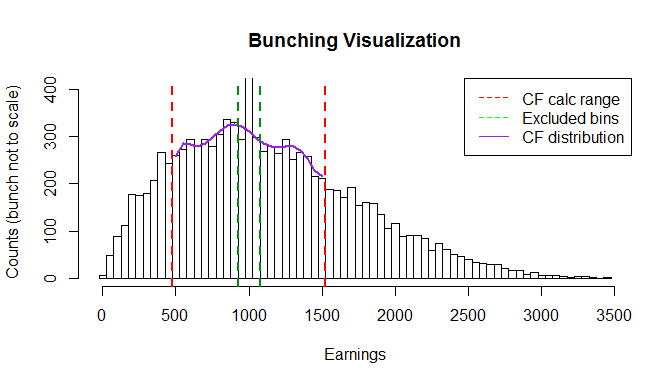kink_est$e ##  0.1662341 quantile(kink_est$booted_e, probs=c(0, 0.05, 0.1, 0.5, 0.9, 0.95, 1))
##         0%         5%        10%        50%        90%        95%
## 0.04387312 0.07718377 0.09195058 0.17941217 0.28779019 0.30870107
##       100%
## 0.39275768

Now, let’s look at a much larger vector, of one million observations. Naturally, the confidence interval generated is much smaller. Note that, with a larger population, we could reliably narrow the bins and get a more accurate estimate.

ability_vec_large <- 4000 * rbeta(1000000, 2, 5)
earnings_large <- sapply(ability_vec_large, earning_fun, 0.2, 0, 0.1, 0, 1000)
kink_est <- bunch(earnings_large, zstar = 1000, t1 = 0, t2 = 0.1, Tax = 0,
cf_start = 50, cf_end = 50,
exclude_before = 5, exclude_after = 5, binw = 10,
nboots = 100, seed = 123)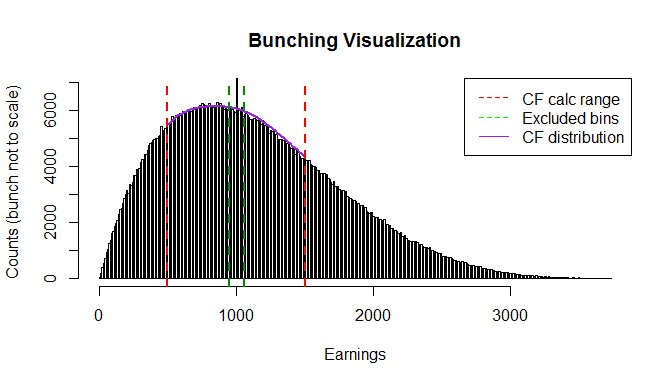kink_est$e ##  0.183655 quantile(kink_est$booted_e, probs=c(0, 0.05, 0.1, 0.5, 0.9, 0.95, 1))
##        0%        5%       10%       50%       90%       95%      100%
## 0.1688440 0.1742967 0.1772704 0.1850824 0.1928552 0.1942905 0.1970645

In fact, with a larger population, we could reliably narrow the bins and get a more accurate estimate. Using only 10 bins before and after the bunching bin:

kink_est <- bunch(earnings_large, zstar = 1000, t1 = 0, t2 = 0.1, Tax = 0,
cf_start = 50, cf_end = 50,
exclude_before = 1, exclude_after = 1, binw = 5,
nboots = 100, seed = 123, draw = F)
kink_est$e ##  0.1949573 quantile(kink_est$booted_e, probs=c(0, 0.05, 0.1, 0.5, 0.9, 0.95, 1))
##        0%        5%       10%       50%       90%       95%      100%
## 0.1879073 0.1901380 0.1915570 0.1955756 0.1988548 0.2003031 0.2025746

## Looking for Trouble

Generally speaking, the estimation strategy in these procedures are likely to attenuate, rather than exacerbate, the estimates for elasticity. However, it is worthwhile to see what happens if, by mistake, we specify the wrong earnings or elasticity.

We can simulate an earning vector with no bunching and see the estimates, and run bunch on it.

earning_vec <- sapply(ability_vec, earning_fun, 0.2, 0.1, 0.1, 0, 1000)
bunch_viewer(earning_vec, 1000, 10, 10, 1, 1, binw = 50, trimy = F)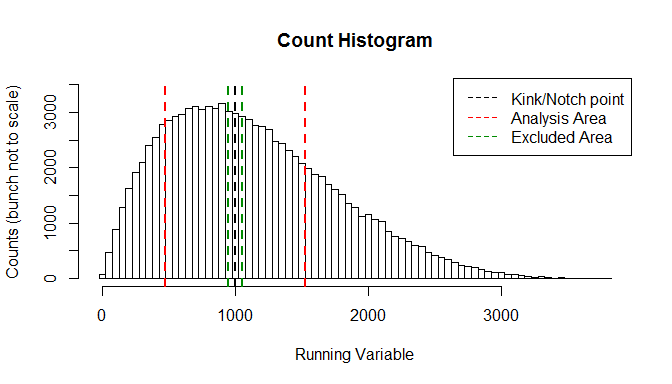kink_est <- bunch(earning_vec, zstar = 1000, t1 = 0.1, t2 = 0.1, Tax = 0,
cf_start = 50, cf_end = 50,
exclude_before = 1, exclude_after = 1, binw = 10,
nboots = 100, seed = 123, draw = F)
## Error in kink_estimator(earnings, zstar, t1, t2, cf_start, cf_end, exclude_before, : No change in marginal tax rate - can't calculate elasticity!

Woops! Can’t do this. The formula for elasticity includes the logarithm of the proportion of net-of-tax rates before and after the kink. Setting them equal generates a NaN, as the zeroed logarithm term is in the denominator.

But let’s see what bunch does when we try to estimate a marginal tax rate change, when in fact there was no change. Presumably, as there is no visible bunching, the estimates would be zero. Indeed:

kink_est <- bunch(earning_vec, zstar = 1000, t1 = 0, t2 = 0.1, Tax = 0,
cf_start = 50, cf_end = 50,
exclude_before = 1, exclude_after = 1, binw = 10,
nboots = 100, seed = 123, draw = F)
kink_est$e ##  0 quantile(kink_est$booted_e, probs=c(0, 0.05, 0.1, 0.5, 0.9, 0.95, 1))
##          0%          5%         10%         50%         90%         95%
## 0.000000000 0.000000000 0.000000000 0.000000000 0.001648733 0.003649318
##        100%
## 0.008581313

What would happen if bunching does occur, but we did not specify the tax rates correctly when running bunch? Let’s create an earnings vector with a tax rate change from 0% to 30%, but run bunch for a smaller tax change, 0% to 20%. As the observed bunching is higher than what would be expected for a change from 0% to 20% with elasticity of 0.2, bunch estimates a higher elasticity of earnings. This kind of user-induced error cannot be detected, so make sure to input the parameters correctly.

earning_vec <- sapply(ability_vec, earning_fun, elas = 0.2, t1 = 0, t2 = 0.3,
Tax = 0, zstar = 1000)
kink_est <- bunch(earning_vec, zstar = 1000, t1 = 0, t2 = 0.2, Tax = 0,
cf_start = 50, cf_end = 50,
exclude_before = 1, exclude_after = 1, binw = 10,
nboots = 100, seed = 123, draw = F)
kink_est$e ##  0.2947799 quantile(kink_est$booted_e, probs=c(0, 0.05, 0.1, 0.5, 0.9, 0.95, 1))
##        0%        5%       10%       50%       90%       95%      100%
## 0.2768846 0.2869495 0.2881759 0.2964715 0.3046559 0.3057848 0.3113277

## Comparison with Existing Packages

To my knowledge, no other R package for bunching analysis is publically available at the time of release of this current version. Chetty et al (2011) did distribute their Stata code, which was used for kink analysis, and I will use it to validate bunchr for kinks. Unfortunately, I cannot use it with the original administrative data used by the researchers. However, I can run a simulation and test for similarity in results. The Stata program was written by Tore Olsen, and can be found in Raj Chetty’s website. To replicate these results, you will need a copy of Stata of course. Download and install the bunch_count function for Stata, and define the working directories properly.

First, I create a dataset with bunchr. The Stata function requires collapsed, binned data. I use bunch_viewer to create and export that. Note that bunch_viewer will create the histogram by placing the kink point in the middle of a bin.

set.seed(1982)
ability_vec <- 4000 * rbeta(100000, 2, 5)
earning_vec <- sapply(ability_vec, earning_fun, 0.3, 0, 0.2, 0, 1000)
data <- bunch_viewer(earning_vec, zstar = 1000, binw = 50, report = T)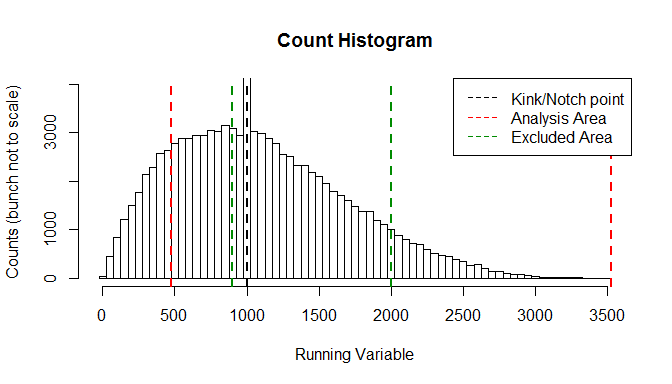sim_data <- data.frame(cbind(data$mids,data$counts))
colnames(sim_data) = c("earnings","counts")

Now, save the simulated earning histogram in comma delimited file, which is easy to read in Stata:

write.csv(sim_data, file="sim_data.csv", row.names = F)

Now, run this code in Stata:

insheet using "sim_data.csv", clear
bunch_count earnings counts, bpoint(1000) ig_low(-10) ig_high(10) low_bunch(-1) high_bunch(1) plot(1) binwidth(50)
outsheet using "compare_results.csv", replace delim(",")

This will generate a plot, output some estimates, and change the dataset so the estimated counter-factuals are included as the plotabc3 variable. Then, the data will be exported as a .csv file which we will import back to R.

chetty_res <- read.csv(file = path)
chetty_res <- chetty_res[order(chetty_res$earnings), ] Now, we can run bunch on the data. I use the same specification regarding the bin width, counter-factual area, excluded area, and correcting for shift on the right side of the notch. The main difference is that, since bunch calculates the estimated elasticity, it needs the marginal tax rates as inputs (this will not matter for the counter-factual estimation). Also note that I shut down the default option for model selection for the counter-factual (mostly useful for notches, and non-existing in bunch_count), and use the default $$7^{th}$$ degree polynomial. estim <- bunch(earning_vec, zstar = 1000, t1 = 0, t2 = 0.2, Tax = 0, cf_start = 10, cf_end = 10, exclude_before = 1, exclude_after = 1, binw = 50, max_iter = 200, correct = T, select = F, poly_size = 7, draw = F) # creating comparison data-frame bunchr_res <- estim$data
comp_data <- cbind(bunchr_res, chetty_res)
comp_data$cf_diff <- comp_data$cf_counts - comp_data$plotabc3 comp_data$per_diff <- 100 * comp_data$cf_diff / comp_data$plotabc3

show_data <- comp_data[,c(1,2,3,9,10,11)]
colnames(show_data) <- c("earnings", "counts",
"bunchr_cf_counts", "bunchcount_cf_counts", "diff",
"percent_diff")

# write.csv(show_data, file="show_data.csv", row.names=F)

# plot the results
plot(show_data$earnings, show_data$counts, type="h",
main="comparing bunching counter-factuals",
xlab="earnings", ylab="counts",
xlim=c(200,2000))
points(show_data$earnings, show_data$bunchr_cf_counts, col="red", pch="*")
points(show_data$earnings, show_data$bunchcount_cf_counts, col="blue",pch="*")
legend("topleft", lty=c(1, NA, NA), pch=c(NA, "*","*" ), col=c("black","red","blue"),
legend=c("real counts","bunchr cf", "bunch_count cf"))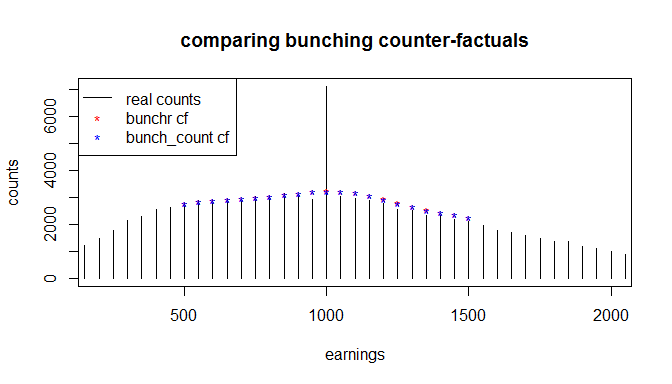sim_data.csv, compare_results.csv and show_data.csv are available in the extdata folder of the installation.

1. Kleven, H J (2016). “Bunching”, Annual Review of Economics, 8(1).

2. Saez, E. (2010). “Do taxpayers bunch at kink points?”

3. Chetty, R., Friedman, J., Olsen, T., Pistaferri, L. (2011). “Adjustment Costs, Firm Responses, and Micro vs. Macro Labor Supply Elasticities: Evidence from Danish Tax Records”, Quarterly Journal of Economics, 126(2).

4. Kleven, H.J., Waseem, M., “Using notches to uncover optimization frictions and structural elasticities: Theory and evidence from Pakistan”, The Quarterly Journal of Economics, 128(2)